# Using of Best Plane Method (B.P.M.) to Estimate the Optimum Number with Value and Direction of Slopes for Farm Units in Irrigation Project

Using of Best Plane Method (B.P.M.) to Estimate the Optimum Number with Value and Direction of Slopes for Farm Units in Irrigation Project
Ammara Technical Institute, Southern Technical University, Maysan 62001, Iraq

Al- Mussaib Technical Institute, Al-Furat Al-Awsat Technical University, Hillah 51009, Iraq

Corresponding Author Email:
inm.wsm@atu.edu.iq
Page:
523-531
|
DOI:
https://doi.org/10.18280/ijdne.150409
16 May 2020
|
Accepted:
25 June 2020
|
Published:
31 August 2020
| Citation

OPEN ACCESS

Abstract:

This research aims to find the number of farm units and the distance between each other with the best direction of slops in the units of irrigation for the previously executed irrigation project. The best plane method (B.P.M.) was chosen in this study. As we knew, this method does not give the correct value of slope when the collector drain canal lies at a higher level than the watercourse canal in irrigation units. (B.P.M.) gives the magnitude for the inverse direction of slope and the required inverse cross irrigation slope. Therefore, it is important to find a solution for this problem by using the conditions and limitations in determining the required slope values of cross irrigation and in the correct direction for irrigation slope to give the low quantity for both cuts and cut to fill ratio, to obtained minimizing in cost.

Keywords:

farm unit, irrigation project, cut - fill ratio, watercourse, optimum slope

1. Introduction

Planning for any project of an irrigation - drainage network needs initially to perform a topographical investigation to prepare the general plan for the network (layout) where the irrigation canal is located at the contour lines with high natural levels while the drain canals are located on contour lines with low levels . After preparing the general plane, the process of designing the sections of canals drains, and installations of all kinds such as regulators, culvert and escapes, and the process of implementing the civil works for the network, then the final and important stage is reached, which is the process of reclamation of agricultural land, which includes two parts of process. The first part of process is leveling and grading the land, which aims to scale and smooth the surface of the earth to obtain a suitable surface for the efficient use of irrigation water and to get rid of excess surface water easily. As what is required in the graduation of the land is not to make the land level, but rather to graduate it with inclines or regular slopes towards irrigation and the perpendicular direction to irrigation .

The grading process involves removing part of the elevated sites to dictate part of the low sites, and in other cases, large quantities and depths should be dug and then leveled. The process of leveling the ground usually requires moving quantities of soil to the number of hundreds of meters. In addition, finishing the flat surface is part of the leveling process, to reduce and limit secondary winding in the farm. The leveling may be preliminary, without accuracy, and for a part of the land that includes removing plateaus and solutions to fill the small depressions in the farm. In this case, there is no need for design planning for the staging as well as the lack of need to divide the farm and its relationship to the number of farm turnouts and the need to install surveys and design levels, but rather be done depending on the consideration to obtain the desired surface of the farm. This leveling may only be necessary for several sprinkler irrigation systems, but it does not fulfill which means the inaccurate leveling, the purpose of the methods of surface irrigation and the reason is that most of requiring a careful leveling of the land . About leveling and grading operations that use surface irrigation methods , the land must be divided into farms, and then the surveys and design levels for the grading work will then be installed. As the second part of process is the land reclamation process, it includes designing and implementing farm drains, and then the agricultural land becomes ready for the process of leaching the soil and rid it of salts.

The (BPM) method that is used to find the optimum slopes in the work of levelling and grading for farm lands depends on being the point of origin for irrigation or levelling directions starting from the high levels area which is the area of farm channel towards the area with low levels which is collector drain . The problem with some of the implemented projects is that the farm channel was implemented in areas with low levels and the collector drain was implemented in areas of high levels. In this study, the modification was made to (BPM) method to solve the problem of the correct direction of irrigation and the preparation of corrective levels of farms to achieve the lowest cut-fill ratio to minimize the cost without affecting the root zone area of plants. Another problem that was observed in the distribution of farm outlets along the farm channel and their relation to the size of the farm, as well as those related to farm drains. Both of which are related to the process of levelling and grading the region. This study will provide solutions to these problems including the distribution of farm outlet and farm drains and the farm size, which achieves irrigation efficiency.

2. Material and Methods

2.1 Study area

Table 1. Values of “longitude and latitude" for study area

 ID E N 1 606,303.956 3,599,149.715 2 603,294.315 3,593,163.505 3 601,442.228 3,591,708.294 4 608,585.992 3,588,632.506 5 621,272.788 3,577,083.421 6 627,702.176 3,582,798.432 7 625,082.796 3,585,457.500 8 624,447.794 3,589,227.820 9 621,828.414 3,593,593.454 10 617,065.90 3,593,990.320

## 1a.png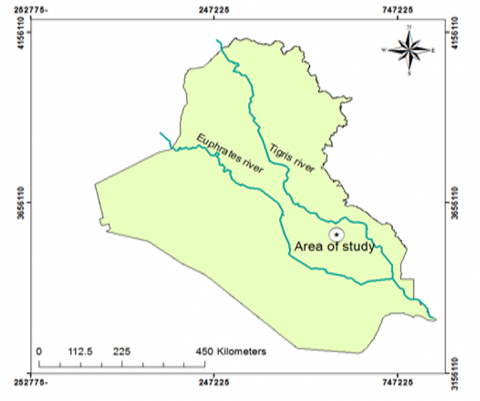(a)

## 1b.png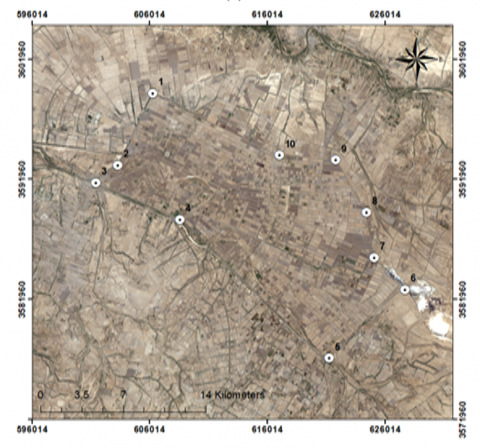(b)

Figure 1. (a) Area of case study; (b) Area of case study

The study area is located in the Sheikh Saad district, located to the southeast of Kut city, which is 50 km away from it in southern Iraq. The study area lies within the limits of longitude and latitude shown in Table 1 along Land sat 8 track (38) path and (167) row (USGS). The location illustrated in Figure 1. This region is located in the central region of Iraq, lands for this region have slightly to moderate slopes. The project depended on the surface irrigation system to irrigate the lands. problems arose in the preparation of designs for the grading and levelling of farms, which are that the watercourse channel was implemented on low-level lands and the collected drain located in high-level lands, therefore it was taken as a study area in this research.

2.2 Boundary of net area for leveling and grading works

When designing leveling and grading inclinations, as well as when carrying out leveling work for half irrigation units, the net area for leveling and grading is initially determined, and it’s based on the distributor canal, watercourse, branch drain, and collector drain reservation  to facilitate agricultural operations, land maintenance and movement of agricultural machinery, Figure 2 .

## 2.png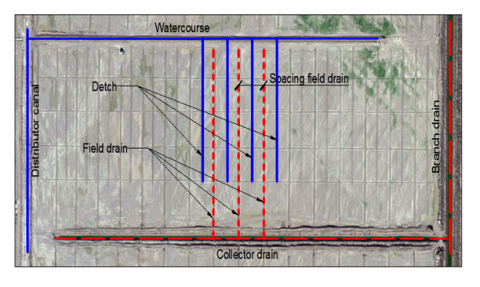Figure 2. Boundary of net area for leveling and grading works

2.3 Locations of field drains and spacing

The spacing distance between field drains is determined according to several methods, such as “Hooghoudt¢s Eq. , Ernst Eq.  and Generalized - Throughout - Ernust Eq. " but how far is the field drain from the distributor canal? What is the distance of the field drain near the branch drain from the branch drain? In addition, what is the distance of upstream of the field drain from the watercourse? These distances are determined according to the following limitations:

(1) The distance between the boundary of landing on the one hand distributor canal and the field drain that near to the distributor canal should not be more than half of the design spacing (L for field drains), whatever the distance of the spacing.

(2) Distance between the end of field drain (upstream) and the centerline of watercourse must be 15 m, Figure 3.

(3) Distance between field drain and center line of branch drain (Le) should not exceed the designed spacing distance plus 30 m, and it should not be less than the designed spacing distance of the field drains, Figure 3.

(4) Field ditch should locate in the middle of the spacing distance for designed field drains, and the distance between the field ditch and collector drain must not be less than 10 m.

2.4 Farm boundaries

The determination of boundary for one farm that is equal to one or more leveling units are depending on the leveling boundaries (reservation) . As well as based on the distribution of field drains and their limitations, and also to the size of the farm unit (as shown in Figure 3).

## 3.png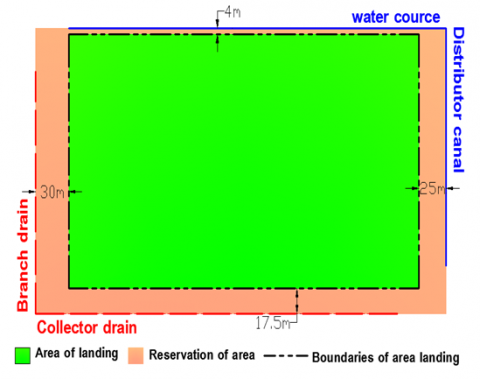Figure 3. Farm boundary

2.5 The optimum slopes for land leveling

The efficiency of irrigation is related to the extent of homogeneity and uniformity of the soil surface so that it is free from undulation that affects the degree of water distribution within the irrigated area, which requires a complete leveling of the soil surface when choosing one of the methods of surface irrigation . The acceptable limits for land leveling and gradation depend on the method of irrigation, soil type and the consideration of erosion and sedimentation process . In this research, the method of basins irrigation was adopted, as the limits of inclination towards irrigation range between (0.04% - 0.15%) and the vertical inclination for irrigation should not be more than (0.04%).

There are many methods by which the design level slope is calculated in the direction of irrigation and in the vertical direction of the irrigation, which is called transverse inclination, these methods are "the best plane method, The average profile method, and Average slope method" . The most accurate method is the plane of the best-fit method, which depends on the least-squares method shown in the following equation, which represents the sum of squares of deviations between the natural levels and the design levels of the grid points.

$S=\sum_{i=1}^{m} \sum_{i=1}^{n}\left[H_{i, j}-\left(a+b X_{i}+c Y_{j}\right)\right]^{2}$     (1)

where,

S=the sum of the squares of the deviations, b = the slope of the plane toward the X-axis, c = the slope of the plane toward the Y-axis, a = constant represents the height of the design plane at the origin point to find the best values for the slopes b and c that give the lowest value to the sum (S), conventional methods can be used in optimal solutions using three equations each representing the partial derivation of Eq. (1) concerning a and b and c with the right-side equation for each of these equations at zero, that is:

$\frac{\partial S}{\partial a}=0, \frac{\partial S}{\partial b}=0, \frac{\partial S}{\partial c}=0$     (2)

By solving the immediate linear equations in Eq. (2), the best values for the coefficients a, b, c are obtained that determine the properties of the design plane, where b is SX represent the slope of the optimum plane towards the X- axis and c is SY. The values of SX and SY resulting from solving the three equations are as follows:

$S_{X}=\frac{\sum_{i=1}^{m}\left[\left(\sum_{j=1}^{n} H_{j}\right) * i\right]-X_{c} *\left[\sum_{j=1}^{n} \sum_{i=1}^{m} H_{i, j}\right]}{\sum_{i=1}^{m}\left[N_{i} * i^{2}\right]-\left[\sum_{i=1}^{m} \sum_{j=1}^{n} N_{i, j}\right] * X_{c}^{2}}$        (3)

$S_{Y}=\frac{\sum_{j=1}^{n}\left[\left(\sum_{i=1}^{m} H_{i}\right) * j\right]-Y_{c} *\left[\sum_{j=1}^{n} \sum_{i=1}^{m} H_{i, j}\right]}{\sum_{j=1}^{n}\left[N_{j} * j^{2}\right]-\left[\sum_{i=1}^{m} \sum_{j=1}^{n} N_{i, j}\right] * Y_{c}^{2}}$        (4)

$\sum_{j=1}^{n} \sum_{i=1}^{m} N_{i, j}$= The total number of farm cells (meshing)

$\sum_{j=1}^{n} \sum_{i=1}^{m} H_{i, j}$= The sum of all levels of farm cells (meshing)

$\sum_{i=1}^{m} H_{i}$=The sum of the levels of column i cells in a direction parallel to the Y- axis

$\sum_{j=1}^{n} H_{j}$=The sum of the levels of column j cells in a direction parallel to the X- axis.

Ni=number of cells in column i, NJ=number of cells in column j, XC=distance from the center of the shape to the Y-axis with a horizontal interval according to the interlacing, YC=distance from the center of the shape on the X-axis with a vertical interval according to the interlacing.

3. The Aim of Study

3.1 The problem of the study

Through the investigations carried out to the Sheikh Saad lands reclamation project, two problems were observed. The first problem, It was noted that some watercourses are located at low ground levels and the reason for this may be the age of the design of the general layout of the irrigation and puncture network that did not occur after topographical changes occurred in the region due to random cutting of the soil by some for material gain, and the other reason is the use Contouring map with a large period when preparing the general plan for the network so that it does not give a clear picture of the ground levels. Thus, if one of the methods used to find the optimum tendencies is used, it gives inclination opposite to the required irrigation direction in the sense that it gives an opposite direction to the irrigation source, and the need for the values of these inclinations is also negated, and if they use arbitrary inclinations, they give very large cut quantities and therefore we do not obtain the design level optimum. The second problem is the ill-considered distribution of farm turnout stations, and therefore this leads to an ill-considered distribution of farm units and the occurrence of variation in their areas so that the efficiency of immersion irrigation decreases and the length of the farm canal increases causing losses in water in addition to an increase in the cost of the project this is because there are more farm turnout than required.

3.2 The proposed solutions

3.2.1 Number of farm turnout

The proposed solution to the first problem is by noting the limits of leveling boundary, the locations of field drain, and the area of the farm unit, it is suggested that the division of farm units and the distribution of farm turnout as shown in Figures 4-a and 4-b, the dotted line represents the leveling boundary for each farm and should be away from Farm turnout with a distance equal to half the distance of the spacing between the designed field drains, while the dashed line represents the leveling boundary of the total area. According to this distribution, farm turnout stations are as shown in Table 2, while farm boundary stations are as shown in Table 3.

## 4a.png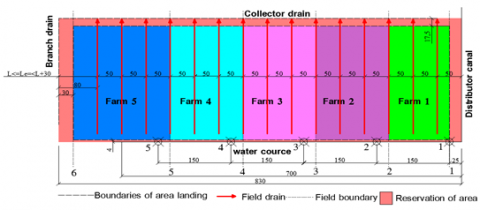(a)

## 4b.png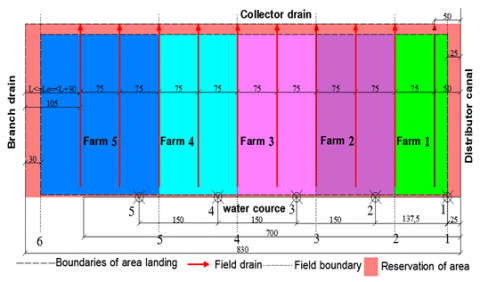(b)

Figure 4. (a) The proposed farm (field) outlet distribution, if the spacing distance between the farms drains is 50 m; (b) The proposed farm (field) outlet distribution, if the spacing distance between the farms drains is 75 m

Table 2. Stations of proposed farm turnout

 Number of Farm turnout Distance of farm turnout from the center line of distributed canal, spacing distance (50 m) Distance of farm turnout from the center line of distributed canal, spacing distance (75 m) 1 25 m 25 m 2 175 m 162.5 m 3 325 m 312.5 m 4 475 m 462.5 m 5 625 m 612.5 m

Table 3. Stations of proposed farm boundary

 Number of Farm turnout Distance of farm boundary from the center line of distributed canal, spacing distance (50 m) Distance of farm boundary from the center  line of distributed canal, spacing distance (75 m) 1 25 m 25 m 2 150 m 125 m 3 300 m 275 m 4 450 m 425 m 5 600 m 575 m

3.2.2 The suggested method for choosing the optimum slopes

(1) Water courses location, when looking at the layout for any implemented irrigation and drainage network, it is observed that there are four cases for the location of the water source, which is the water courses, as shown in Figure 5. The dark point is the point of origin and it is the meeting point of the watercourses canal with distributor canal. It represents the direction of irrigation and the point of origin has the highest design level in the plane of horizontal and vertical inclination. This point is fixed, meaning that the direction of irrigation cannot be changed, because the farm turnout was built previously.

(2) When using the best plane method to find the optimum slopes for the design level, it naturally takes the natural slope of the land, but the problem as I have already mentioned is that the watercourses are located in a low-level land and the collector drain is in a land with high levels and therefore the origin point may be in one of the other three corners and this causes a change in the irrigation direction at point( B, C or D), and it is assumed that the origin point has the highest level at point (A) as shown in Figure 5.

To solve this problem, subroutine programs have been prepared showing how to choose the optimum slopes that give the lowest amount of both cut and the cut-fill ratio, and below is an explanation of the sub-programs for choosing the optimal slopes and for the first case. The subprograms include the main subprogram and four other subprograms are A, B, C, and D. In the main subprogram, the true slopes of SX and SY are found in the best plane method, and based on the signals of these slopes, process for one of the four subprogram's is used to correct the direction of slope and find the lowest amount of cut possible according to the following conditions:

(1) If SY and SX are negative then we will move to sub-programs A, which explains later.

(2) If the SY signal is positive and SX is negative then we will move to sub-programs B, which explains later.

(3) If the SY signal is negative and SX is positive, then we will move to sub-programs C, which explains later.

(4) If the SY and SX signal is positive then we will move to sub-programs D, which explains later.

## 5.png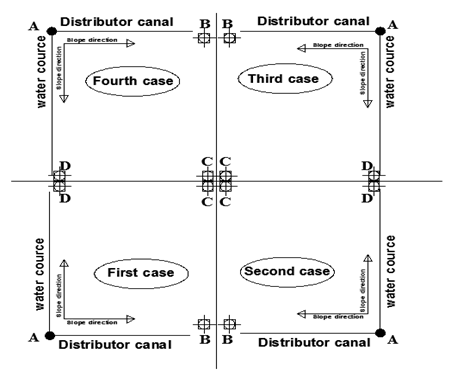Figure 5. The four cases of the direction of the irrigation source

Subroutine (A)

When the SX, SY signal is negative in this subprogram, the following processing operations are performed:

(1) Initially, the slope value of the SX is compared with the slope determinants that range from 0.15% to 0.04%. If the slope value is greater than the highest value of the slope determinants, the upper limit of the slope determinants is chosen, and if the slope value is less than the lowest value for the slope determinants, a choice is made The minimum slope determinants, while if the slope value is a value between the upper and lower limit of the slope determinants, its value, which is found by the best plane method, is chosen because it is within the limit of the slope values.

(2) Then the value of slope for SY is compared with the limitation of slopes in which range from 0.025% to 0.04%. If the slope value is greater than the highest value of the slope determinants, the upper limit of the slope determinants is chosen, and if the slope value is less than the lowest value of the slope determinants, the limit is chosen. The minimum slope determinants, while if the slope value is a value between the upper and lower limit of the slope determinants, then the value that was found is determined by the best plane method because it is within the limit of the slope values.

(3) After the slope values have now been chosen, the value of the normal levels will be transferred according to the slopes adapted to the point of origin, and then find the other values of the design levels for the farm.

Subroutine (B)

When the SY signal is positive and the SX signal is negative in this subprogram, the following processing operations are performed:

(1) Initially, the slope value of the SX is compared with the slope determinants that range from 0.15% to 0.04%. If the slope value is greater than the highest value of the slope determinants, the upper limit of the slope determinants is selected, and if the slope value is less than the lowest value for the slope determinants, a choice is made. The minimum slope determinants, while if the slope value is a value between the upper and lower limit of the slope determinant, its value, which is found by the best plane method, is chosen because it is within the limit of the slope values.

(2) Here, since the SY signal is positive, the minimum slope limits are equal to 0.025%.

(3) After the slope values have now been chosen, the value of the normal levels will be transferred according to the slopes adapted to the point of origin, and then find the other values of the design levels for the farm.

Subroutine (C)

When the SY signal is negative and the SX signal is positive in this subprogram, the following processing operations are performed:

(1) The inclination value of SY is first compared to the inclinometer determinants that range from 0.025% to 0.04%. If the inclination value is greater than the highest value of the inclination determinants, the upper limit of the inclination determinants is chosen, and if the inclination value is less than the lowest value of the inclination determinants, the limit is chosen. The minimum slope determinants, while if the slope value is a value between the upper and lower limit of the slope determinants, its value, which is found by the best plane method, is chosen because it is within the limit of the slope values.

(2) Here, since the SX signal is positive, the minimum slope limits are equal to 0.04%.

(3) After the slope values have now been chosen, the value of the normal levels will be transferred according to the slopes adopted to the point of origin, and then find the other values of the design levels for the farm.

Subroutine (D)

When the SY and SX signals are positive in this subprogram, the following processing operations are performed:

(1) Here, since the SY signal is positive, the minimum slope limits are equal to 0.025%

(2) Here, since the SX signal is positive, the minimum slope limits are equal to 0.04%.

(3) After the slope values have now been chosen, the value of the normal levels will be transferred according to the slopes adopted to the point of origin, and then find the other values of the design levels for the farm.

And also, for the other cases. After applying the proposed method to find the optimum slopes, the process of calculating the depths of the cut and fill is done, and thus finding the volume of the cut and fill in several ways, including the method of addition and the method of the four points , after which the comparison process with the cut- fill ratio is carried out. In this research, the ratio has been adopted between (1.15% - 1.30%).

If the ratio of cut to fill is less than the minimum, a process of lowering the level begins to reach the acceptable percentage, but if the ratio of cut to fill is higher than the upper limit, the process of raising the level to reach the acceptable percentage begins and thus obtaining the lowest ratio of cut to fill or the minimum amount of cut possible.

Figure 4 represents half of the irrigation unit, and the spacing distance between the design farm drains is equal to (75m), knowing that all of the determinants mentioned in terms of the sites of farm drains and farm canal and the leveling limits are general for any distance between the farm designers regardless of their value. Note that the watercourse is located at low natural levels, as shown in the field (A). Also, it is noticed that the normal level on the side of the watercourse is 6.20, and on the side of the collector drain is 6.36.

When applying the program using the best plane method to find the value and direction of the optimum slope shown in Table 4, it was observed concerning field (A) that the slope signal toward the X-axis is positive in the sense that the design level of the field center is increasing towards the X-axis and also concerning the slope towards the Y-axis to have it A positive signal also, and this gives the highest level of leveling opposite to the direction of irrigation and not at the point of origin (A) as in Figure 5, then we use the proposed method determinants to find the value and direction of the optimum slope.

In the field (A), since the signal is positive for both slopes, then we use the minimum inclinations according to the proposed program procedure and the change of direction of the inclinations, as well as the same for the field (B). Field (C) notes that the inclination towards the X-axis is positive, then its minimum is chosen, and its direction changes in the required direction.

As for the inclination towards the Y-axis, the signal is negative and the slope value is equal to (0.00778) which is within the approved slope limits, it is adopted according to subprogram procedure (C). For field (D), the inclination toward the X-axis is increased, so the minimum inclination is chosen, while the inclination towards the Y-axis is negative, as can be seen in Table 4. The slope value is equal to (0.0283) and it is greater than the maximum approved inclinations. Therefore, the maximum approved inclinations are chosen according to the procedure of the proposed subprogram (C), and therefore these tendencies give the lowest amount of cut possible.

On the right side of Figure 6, which is the results of the program, we observe the design information using the best plane method in terms of finding the normal levels and the center of the farm. The optimum tendencies are those obtained after using the best plane method with the determinants method.

Also, finding the average value of levels after adjusting the height of the level to achieve the best cut-fill ratio. As for the lower part of the results for each farm, it is a process of comparing the levels of water in the watercourse with the average value of levels of the farm to make sure of the process of flooding the ground to leach the soil.

Table 4. Mechanism for choosing the optimal values and direction of slope

 According to limitation of the proposed method Best Plane Method Value for each 25 m Signal Value for each 25 m Signal Slops Field 0.01 - 0.02103 + Sx A 0.00625 - 0.00488 + Sy 0.01 - 0.02266 + Sx B 0.00625 - 0.00365 + Sy 0.01 - 0.0177 + Sx C 0.00778 - 0.0078 - Sy 0.01 - 0.03056 + Sx D 0.01 - 0.03377 - Sy 0.01 - 0.00939 + Sx E 0.01 - 0.0283 - Sy
4. Result and Discussion

(1) For the first problem, which is farm turnout stations, we deduce what has been presented through the farm unit and to the levelling boundaries illustrated in Figure 2. With based on the proposed farm boundaries shown in Figure 3. And through the determination of the field drains as shown in the Figure 4a, 4b. The location of the first farm turnout should be at a distance half the spacing distance from the levelling boundaries, and the second is half the distance of the spacing distance from the farm boundaries so that the field ditch is in the middle of the spacing distance, and on this basis, the field ditches are located and thus benefit from reducing the length of the field ditches. Likewise, getting rid of the problem of the difference in the levels of levelling between the first and second farms and a homogeneous and equal division as far as possible for the area of farms and the most important thing is to reduce the number of farm turnout. When taking investigations of a hundred and fifty irrigation units implemented in the Sheikh Saad project, it was noted that the watercourse contains six-farm turnout and that the second farm turnout is close to the first farm turnout and is useless and when comparing it with the proposed solution he notes the need for only five-farm turnout. Figure 7 shows the investigations for half of the irrigation units, out of a total of one hundred and fifty irrigation units, shown in Figure 7, with the sign (Ø).

(2) For the second problem, which is to find the optimum slopes, a program has been written using the computer programs incorporating the best plan method in addition to the aforementioned proposed method and compared to the manual solution and the results of the program have been proven correct. This program is distinguished from the programs produced by some universities, for example, a program prepared by the "World Irrigation Information Network" at Utah State University which not considering the direction of irrigation, depending on the assumption that the irrigation is placed in high areas . In the figure it shows the results after the data of the ground levels were entered into the designed program, the first number of each cell represents the ground level of the surface (investigations) and the second number represents the design level after adopting the optimal inclinations (slopes), while the third number in each cell represents the depth of cutting or filling (Noting that the negative sign here in this research means the depth of the cut), while the number marked in bold and underlined, represents the point of origin .

## 6.png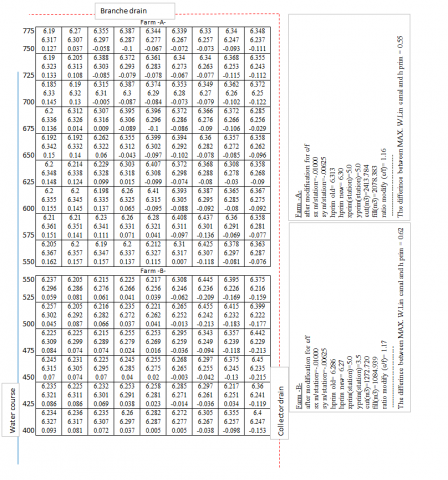## 6b.png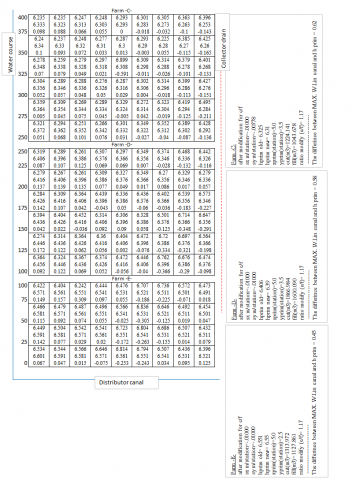Figure 6. Design levels for leveling and grading work for half irrigation units

## 7.png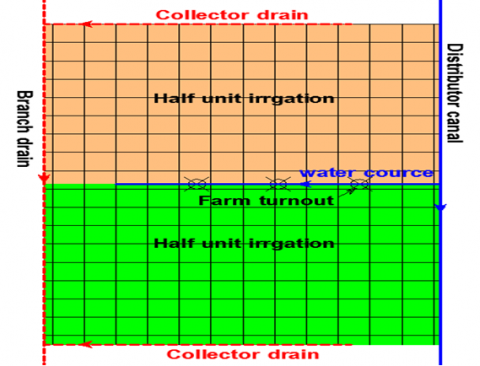Figure 7. Stations of farm turnout for implemented irrigation units

5. Conclusions and Suggestions

(1) The distribution of farm outlets is not arbitrary, but rather the unit of the farm and the spacing distance between the farm drains and the relationship between them, this contributes to determining the number of farm outlets. The efficiency of irrigation is not by increasing the number of these outlets, where one farm outlet is sufficient to irrigate one farm unit and thus reducing the number of outlets, which leads to a reduction in cost.

(2) The distribution of farm outlets according to current research leads to the division of farm by almost equal areas and this helps in the fair distribution of farm to farmers, and also contributes to reducing the difference in levels between one farm and another.

(3) Reducing the quantities of cutting and finding the optimal cutting- filling ratio depends on finding the optimum slopes, where the finding of these slopes has been treated if the watercourse is in the low ground and the collecting drain in the high ground. In addition, reducing the amount of the cutting leads to avoiding damage to the soil layer in which the roots of the plants grow as well to reduce the cost of these projects.

(4) When preparing designs for projects of surface irrigation networks, especially in Iraq, the designer must start with the design of farm units, levelling operations, distribution of farm drains first, and distribution of farm outlets according to it after setting an accurate contour map with a small contour period to avoid placing watercourse in the high ground.

(5) Contour maps must be prepared accurately and use of modern surveying devices and modern programs in their preparation with constantly updated.

(6) The sub-programs that have been prepared can be converted into ready to use programs that can be used during work by linking them to modern levelling machines whose blades work with the laser and thus the levelling of the farms is carried out without the use of pegs.

(7) The levelling operations are very important, especially when conducting the surface drain of soil, as well as leading to irrigation efficiency, and therefore it must be accomplished, but with economic feasibility, that achieves the least amount of cut.

References

 Dawoud, M.A., Ewea, H., Abdel, H. (2011). Sustainable development via optimal integration of surface and groundwater in arid environment: Nile river quaternary aquifer case study. Journal of King Abdulaziz University: Metrology, Environment and Arid Land Agricultural Sciences, 142(575): 1-53. https://doi.org/10.4197/Met.22-3.2

 Xu, L., Dai, F.C., Tu, X.B., Javed, I., Woodard, M.J., Jin, Y.L., Tham, L.G. (2013). Occurrence of landsliding on slopes where flowsliding had previously occurred: an investigation in a loess platform, north-west China. Catena, 104: 195-209. https://doi.org/10.1016/j.catena.2012.11.010

 Albaji, M., Golabi, M., Nasab, S.B., Zadeh, F.N. (2015). Investigation of surface, sprinkler and drip irrigation methods based on the parametric evaluation approach in Jaizan Plain. Journal of the Saudi Society of Agricultural Sciences, 14(1): 1-10. https://doi.org/10.1016/j.jssas.2013.11.001

 Raeisi-Vanani, H., Shayannejad, M., Soltani-Toudeshki, A.R., Arab, M.A., Eslamian, S., Amoushahi-Khouzani, M., Ostad-Ali-Askari, K. (2017). A simple method for land grading computations and its comparison with genetic algorithm (ga) method. International Journal of Research Studies in Agricultural Sciences (IJRSAS), 3(8): 26-38. http://dx.doi.org/10.20431/2454-6224.0308004

 Ertunç, E., Tayfun, C.A.Y. (2017). Determination of Optımum Irrigation Network in Land Consolidation Projects. Journal of International Environmental Application and Science, 12(3): 161-167.

 Nopper, J., Lauströer, B., Rödel, M.O., Ganzhorn, J.U. (2017). A structurally enriched agricultural landscape maintains high reptile diversity in sub‐arid south‐western Madagascar. Journal of Applied Ecology, 54(2): 480-488. https://doi.org/10.1111/1365-2664.12752

 Nagrah, A., Chaudhry, A.M., Giordano, M. (2016). Collective action in decentralized irrigation systems: Evidence from Pakistan. World Development, 84: 282-298. https://doi.org/10.1016/j.worlddev.2016.02.003

 Maisiri, N., Senzanje, A., Rockstrom, J., Twomlow, S.J. (2005). On farm evaluation of the effect of low cost drip irrigation on water and crop productivity compared to conventional surface irrigation system. Physics and Chemistry of the Earth, parts A/B/C, 30(11-16): 783-791. https://doi.org/10.1016/j.pce.2005.08.021

 Armstrong, D. (2010). Modelling dairy farming systems. Technical Bulletin, the State of Victoria, Department of Primary Industries.

 Hassanli, A.M., Ahmadirad, S., Beecham, S. (2010). Evaluation of the influence of irrigation methods and water quality on sugar beet yield and water use efficiency. Agricultural Water Management, 97(2): 357-362. https://doi.org/10.1016/j.agwat.2009.10.010

 Hassanli, A.M., Ebrahimizadeh, M.A., Beecham, S. (2009). The effects of irrigation methods with effluent and irrigation scheduling on water use efficiency and corn yields in an arid region. Agricultural Water Management, 96(1): 93-99. https://doi.org/10.1016/j.agwat.2008.07.004

 Mushi, C.A., Ndomba, P.M., Trigg, M.A., Tshimanga, R.M., Mtalo, F. (2019). Assessment of basin-scale soil erosion within the Congo River Basin: A review. Catena, 178: 64-76. https://doi.org/10.1016/j.catena.2019.02.030

 Prochaska, A.B., Santi, P.M., Higgins, J.D., Cannon, S.H. (2008). Debris-flow runout predictions based on the average channel slope (ACS). Engineering Geology, 98(1-2): 29-40. https://doi.org/10.1016/j.enggeo.2008.01.011

 Masih, I., Giordano, M. (2014). Constraints and opportunities for water savings and increasing productivity through Resource Conservation Technologies in Pakistan. Agriculture, Ecosystems & Environment, 187: 106-115. https://doi.org/10.1016/j.agee.2013.07.003

 World Irrigation Information Network. Land leveler software, users manual, Utah State University. (2005).

 Bhatt, R., Sharma, M. (2009). Laser leveller for precision land levelling for judicious use of water in Punjab. Extension Bulletin. https://doi.org/10.13140/RG.2.1.3688.8806